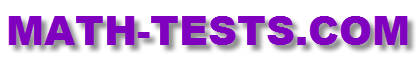#Are you smarter than a fifth grader? Take a 5th grade math test provided on this website to assess your math knowledge for this grade level. The following online quizzes and tests are based on the fifth grade math standards.

These online tests are designed to work on computers, laptops, iPads, and other tablets. There is no need to download any app for these activities.

Identify place value of decimals and whole numbers through millions. Complete multiple choice questions and drag-and-drop activity.

Multiply multi-digit whole numbers to demonstrate understanding of basic multiplication facts.

Convert Customary Units Math Test
Convert customary units such as inches, feet, pounds, gallons, quarts to different units of mearsure. Units of length, mass, and capacity are included.

Convert Metric Units Math Test
Convert metric units such as mass, length, and capacity to different units of measure in these multple choice and drag-and-drop activites. Milliliters, kilometers, kilograms, grams are included.

The Coordinate Plane
Locate points in the coordinate plane and identify their coordinates in this interactive math test activity.

Classify Two-Dimensional Figures Test
Identify two-dimensional figures based on their characteristics in these multiple choice and drag-and-drop questions.

Properties of Multiplication Math Test
Recognize associative, distributive, commutative, and identity properties of multiplication shown in the equations in each question in this online math test.

Division Facts Math Test
Divide numbers up to three digits as you recall division facts up to 12 in these challenging math problems.

Return from the 5th Grade Math Test Page to the Math Tests homepage.